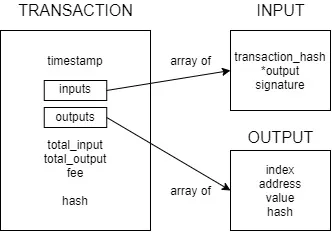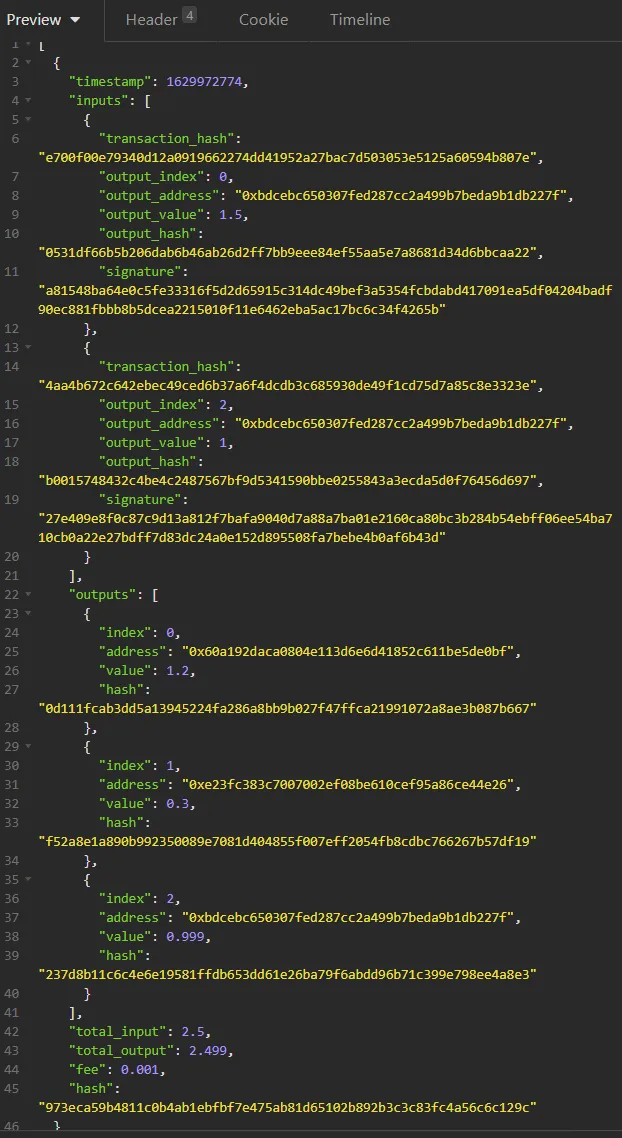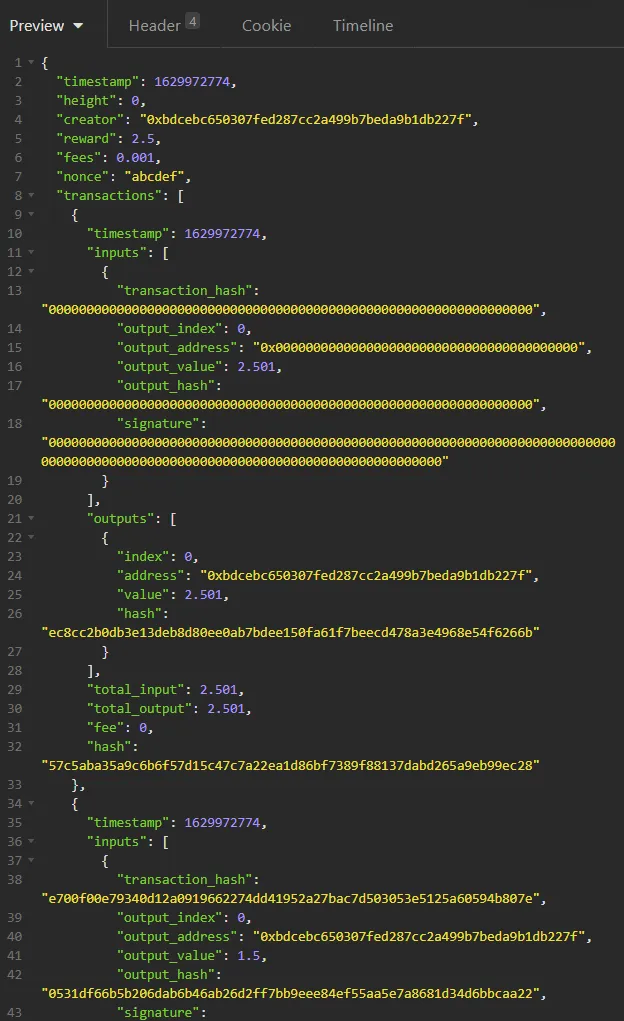Programming - Implementing a Blockchain using Python and Flask [Dev Blog 6][Image 1]

Introduction

Hey it's a me again drifter1!

Well, well, I said that I didn't want to complicate things too much, but tracking spent transactions was way too difficult using the previous transaction format. So, here I am changing transactions to include an array of inputs and outputs. That way, each Input will basically target a previous Output of the Blockchain. Of course, I will not get into the complete code changes, but you should be able to grasp the concept better in the Testing code. The transaction posting endpoint callback will now verify the signature of all the inputs, before posting the transaction. At this point, the UTXO structure that will be implemented later on, will help us check if the Output that is referenced truly exists and if it has been spent. We could even include some form of confirmation check, number of blocks on-top of the block that the transaction resides on, in order to allow only "deep" transactions to be spent. We shall see...

Additionally, in order to make it easier to track the unspent block reward, the first transaction of each block will be the block reward transaction (also known as coinbase transaction). Thus, the block structure has also been tweaked a little, by including the total fees as a new field, and by changing the "reward_address" field into a "creator" field, where the block creator can put anything. For now, still only the block hash is verified by the corresponding endpoint callback. Later on, we will connect the block logic with the UTXO and unconfirmed transactions structures.

So, without further ado, let's get more in-depth!

Reformed Transaction Structure

With the new transaction format, in order to make a transaction one needs to specify previouss outputs in the blockchain that will be used as an inputs. These outputs need to be unlocked, by signing them using the corresponding private key. And the total input value minus some fee for the miner/validator to pick up, can then be sent to new outputs.

The validation of an Output is very easy, as the validator simply has to check if the hashed public key (address) that can be extracted from the {hash, signature} pair is equal to the address referenced in the Output. The exact same Code that we used before for validating the "sender", but with some-what inverted logic.

So, how does the new Transaction look like?Choosing the output to be used as input is as simple as specifying the hash of the transaction the output was in, as well as some info that is useful for locating the exact output. For now its the whole output info, but when the UTXO is ready it will only be the index of the output.

For an output, one simply specified the value to be sent, as well as the receiver's address (the hash of the public key).

Inputs, outputs and the whole transaction can be forged quite easily with lots and lots of helper functions, and the whole from/to JSON format conversion has also been taken care of!

New Transaction Validation Checks

The post transaction endpoint callback now does the following checks:

1. Verify JSON format using json_transaction_is_valid()
2. Recalculate and check total_input and total_output
3. Check if total_input - fee equals the total_output
4. Recalculate and check transaction hash using calculate_transaction_hash()
5. Verify all input signatures by looping through the inputs array and calling verify_signature() on each {signature, hash, output_address} pair
6. Add transaction to local transactions.json if not already in the structure

When the UTXO is ready, it will also be necessary to check if the output that is referenced truly exists and if it has not been spent yet!

Block Structure Tweak

A block is now looking like this:The coinbase transaction doesn't need to contain a valid input, and of course no fee needs to be applied. So, the output value of that transaction will be exactly equal to the static block reward plus the total fees that the transaction posters have submitted.

Shall we burn some fees like Ethereum does since the London Hardfork and EIP-1559?
That should be interesting to implement!

Anyway, that's about it with the important changes in the block...

Now, let's get into an example run...

The testing code now looks like this:

private_key = json_retrieve_private_key(wallet_json)
# create test transaction input0 = Input( transaction_hash="e700f00e79340d12a0919662274dd41952a27bac7d503053e5125a60594b807e", output_index=0, output_address=address, output_value=1.5, output_hash="0531df66b5b206dab6b46ab26d2ff7bb9eee84ef55aa5e7a8681d34d6bbcaa22", ) sign_input(input0, private_key)
output0 = Output( index=0, address="0x60a192daca0804e113d6e6d41852c611be5de0bf", value=1.2 ) calculate_output_hash(output0)
output1 = Output( index=1, address="0xe23fc383c7007002ef08be610cef95a86ce44e26", value=0.3 ) calculate_output_hash(output1)
transaction = Transaction( inputs=[input0, input1], outputs=[output0, output1, output2], total_input=2.5, total_output=2.499, fee=0.001 ) calculate_transaction_hash(transaction)
json_transaction = json_construct_transaction(transaction) local_post_transaction(settings, json_transaction)
# create test block reward_input = Input( output_value=2.501 )
reward_transaction = Transaction( inputs=[reward_input], outputs=[reward_output], total_input=2.501, total_output=2.501, fee=0 ) calculate_transaction_hash(reward_transaction)
block = Block(height=0, creator=address, reward=2.5, fees=0.001, nonce="abcdef", transactions=[reward_transaction, transaction], prev_hash="e700f00e79340d12a0919662274dd41952a27bac7d503053e5125a60594b807e")
calculate_block_hash(block) json_block = json_construct_block(block) local_create_block(settings, json_block)

The test transaction is created in parts. Two inputs and three outputs are created using the corresponding Input and Output classes. Each input is signed using the sign_input() function, and each output hash is calculated using calculate_output_hash(). These inputs and outputs are passed to the Transaction constructor, and the corresponding transaction hash is calculated using the function for that. Lastly, the transaction is turned into JSON format and sent to the local endpoint for validation.

Retrieving the posted transaction using Insomnia, yields:For the block, a new coinbase transaction needs to be defined with basically blank input and the output containing the rewarding address. The output is hashed, the transaction is formed and hashed, and the block is easily constructed by including some more dummy data for the nonce and previous hash. The block hash is calculated, the block is turned into JSON and ready to be sent to the local endpoint.

Retrieving the posted block, yields a big JSON file, which starts out like this:You can easily see the coinbase transaction, which includes a whole lot of 0s. In order to spent that output later on, one would simply have to reference the transaction hash, which is "57c5aba35a9c6b6f57d15c47c7a22ea1d86bf7389f88137dabd265a9eb99ec28", as well as the output index, which is 0, and sign the output hash using the private key. The UTXO is bound to be interesting to implement. I hope that you are all as excited as I am!

RESOURCES:

Images

The rest is screenshots or made using draw.io

Final words | Next up

And this is actually it for today's post!

Now that transactions are in a more Bitcoin-like format, the next logical step should be the UTXO structure. Using such a structure it should be very easy to track the wallet/address balances and to validate the Inputs of transactions...

I will keep you posted on my journey! Expect such articles to come out weekly, or even twice a week, until I'm finished!Keep on drifting!

H2
H3
H4
3 columns
2 columns
1 column